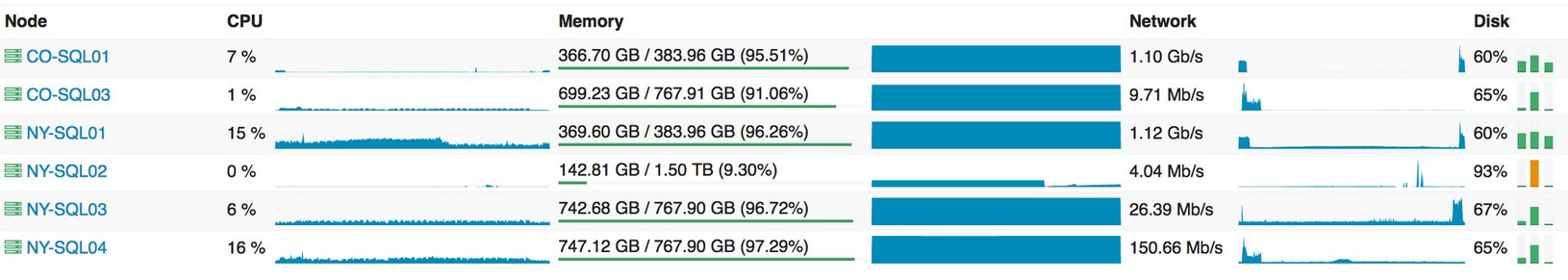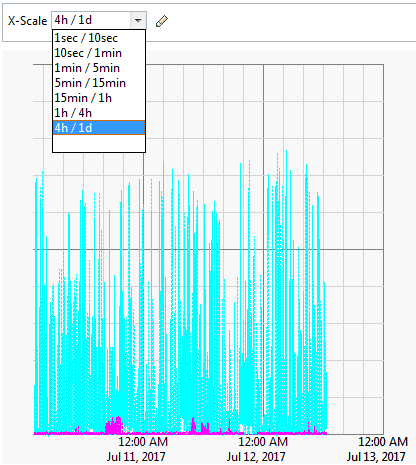# Performance - Waiting Time - Waiting Line (Queue)

A process that waits is also known as queued (waiting to execute).

wiki/Queueing_theory is the mathematical study of waiting lines, or queues

## Model

Waiting times follows an Exponential Distribution in the real world because it's a poisson process

Discover MoreCPU - Wait Time (Queued, Blocked)

The Waiting time performance metrics in the context of CPU. See also: Formula's non-idle wait time != “cluster wait time”+“user I/O wait time”+“concurrency wait time”+“application wait...Collection - Queue (FIFO)

A queue is collection: that follows by default a first-in, first-out methodology where the first element is the first element to be retrieved / removed where the concept of first may be defined....Performance - Saturation (Wait Time|Queue Length)

Saturation is where a resource has more load than it can handle. It can be seen either as: the length of a request queue, or time spent waiting. as a queue length. eg, “the CPUs have an...Process - Poisson Process

The Poisson process is a stochastic process in which events occur: continuously independently (of the time since the last event) - (ie random) at a constant / known average rate in a fixed interval...Statistics - Exponential Distribution

exponential in Distribution exponential distribution is the probability distribution of the time between events in a Poisson process. The value (mostly time) between each pair of consecutive events in...